# Ripple Voltage (unfiltered)

#### CurlsOnKeys

Joined Jan 3, 2015
47
Hi all,

after lots of reading, Googling and ending up getting the same results over and over again, I decided to take a shot and ask the question here.

I'm trying to wrap my head around how a rectified waveform is actually the sum of an AC and DC component. I'm taking an example of a half wave rectifier and I'm talking about the unsmoothed ripple here, so no filter capacitor included.

Let's say I have a maximum secondary voltage Vp2 of 50V, applied to a load resistance of 800 ohms. The resulting maximum current Im is 50V/800, or 62.5 mA.
Idc then equals 62.5/pi (half-wave rectifier) or 19.89 mA. Irms equals 62.5/2 = 31.25 mA.

Now, a rectified current (or voltage) is actually the sum of an an AC current + DC current. Let's say I visualise the above example (see picture). If I understand it correctly, the peak-peak value of the AC component is equal to the peak-value of the rectified wave (62.5 mA in this example). The minimum value of the AC component however is (in this example) -19.89 mA, so if you add the 19.89 mA DC-component to the AC-component, you get a nice rectified result (blue waveform).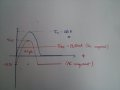The way I understand it and cross-checked with examples and books (Principles Of Electronics among one of them), this drawing makes sense.

However, there's also the maths. As stated in various sources and books: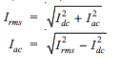Iac, being the rms value of the ripple current.
Now if I make that calculation in my example (sqrt of 31.25² - 19.89²), I get an Iac(rms) of 24.10 mA (as extra proof I calculate the ripple factor Iac(rms)/Idc = 24.10/19.89 = 1.21, which is ripple factor for a half wave rectifier, so this adds up).

However, when I try to calculate the Iac(peak) value out of the rms, I get 24.10 mA x 2 (half wave rectifier), which give me 48.20 mA.
Which is different from the peak value I got when I made the drawing (should be the same

Now I know I'm doing something wrong and since the math works out, it probably is the drawing, but if that is not correct, can someone explain me how exactly the rectified output is a combination of AC and DC? Because when I draw it this way (which somehow seems to make sense, the math doesn't work out?

Thanks!

#### MikeML

Joined Oct 2, 2009
5,444
If ever there is a case for simulation, this is it:

Here is your situation with an ideal voltage source (which no transformer will ever be), ideal Silicon rectifiers (where real rectifiers model resistances and capacitances), with zero resistance wires...

I start with a 50Hz sinusoidal voltage source of 100Vpp. Note that the forward conduction voltage drop across the two forward-biased Si rectifiers causes the peak value of V(o) to be only 48.47V.

I let LTSpice integrate V(o) to show that the time-averaged DC value of V(o) = 30.36V and its Root Mean Square (effective) value is 34.00V.

Note that the voltage measured at V(o) with a voltmeter would read 30.36Vdc.

Note that the power dissipated (heating) in R1 would be Erms^2/R1 = 1.445Watts

Confused yet?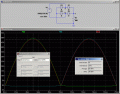Last edited:

#### MikeML

Joined Oct 2, 2009
5,444
Last edited:

#### CurlsOnKeys

Joined Jan 3, 2015
47
Hey Mike,

Confused, no, not about your calculations, yes, in the sense that I don't really understand what this has to do with my original questionYou simulated a full wave rectifier, I used an example with a half wave rectifier. And you're right, I ignored the voltage drop over the diodes, since that wasn't really part of my question. I was just trying to grasp why the measurements in a drawing of what seemed to be the AC component of the rectified voltage didn't correspond with the math.

I perfectly understand your example, V(o) is 48.47V, so you take .765V PD over the diodes, Vrms is 48.47/sqrt2 = 34.00 (I get 34.27 due to round-off error) and Vdc (which you wil measure with a voltmeter) is 2xVo/pi = 30.36 Vdc (I get 30.85, also due to round-off errors).

Let me try to formulate it a bit different. If you have constant DC voltage of 30.85 Vdc (to continue on your example) and you have a peak rectified voltage of 48.47V, you can theoretically "build" this is with an AC voltage of the same peak-value (48.47V) that has a maximum positive value of (48.47 - 30.36) = 17.62V (and a minimum of 17.62 - 48.47 = -30.85) and to this AC voltage you add your 30.85V DC voltage. This wil "shift" the AC voltage all the way up ("rectifies" it) to what you eventually become: a DC component + an AC component. Of course, in practice you don't do it this way, I get that, but I'm trying to understand what exactly is your "Vripple(rms)"?
Because as I see it, the AC-component has the exact same peak-peak (and thus rms value) as V(o), with the difference that it's not DC shifted. So how come the Vrms(ripple) you calculate (for full wave rectifier Vdc x 0.48) is different from V(o)rms? We're talking about the same waveform, only not DC-shifted, no?

Where in my reasoning am I wrong?

If ever there is a case for simulation, this is it:

Confused yet?

View attachment 94493

#### wayneh

Joined Sep 9, 2010
17,189
Those simplified formulas - V/π or V/2 - apply to the case where the flat part of the waveform is at zero voltage. You cannot use the simplified result when that's not true. As Mike beat me to it, you have to do the integral.

#### PeterCoxSmith

Joined Feb 23, 2015
148
You should do a Fast Fourier Transform of your waveform to get the spectrum, then you can say that the waveform = the sum of many AC components plus the DC as extracted by the FFT. Your waveform is not the sum of a single AC waveform and a DC level.

#### CurlsOnKeys

Joined Jan 3, 2015
47
You should do a Fast Fourier Transform of your waveform to get the spectrum, then you can say that the waveform = the sum of many AC components plus the DC as extracted by the FFT. Your waveform is not the sum of a single AC waveform and a DC level.
Hi Peter,
thanks for your input. I get that. As a matter of fact I have a course in which an FFT is given for a half wave rectified voltage with a DC value of (almost) 0.3V. I plotted the series here without the DC, so what I seem to get (after summation) is one single AC waveform (which makes sense, no? The FFT shows the individual frequencies, but in the end you get one waveform?). Add to this wave the constant DC of 0.3V and you get the rectified wave.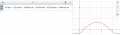Those simplified formulas - V/π or V/2 - apply to the case where the flat part of the waveform is at zero voltage. You cannot use the simplified result when that's not true. As Mike beat me to it, you have to do the integral.
Thanks Wayneh; ok I get that. But is it right to say that the AC component has the same peak-peak value and is basically the same waveform as the final Vout, it's only not DC shifted? As is shown in various textbooks and here also: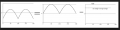.
The RMS value of the final Vout and the AC component differ though, because of what you say, namely the fact that the flat part of the AC is not at zero? Correct?

#### The Electrician

Joined Oct 9, 2007
2,914
However, when I try to calculate the Iac(peak) value out of the rms, I get 24.10 mA x 2 (half wave rectifier), which give me 48.20 mA.
Which is different from the peak value I got when I made the drawing (should be the same

Now I know I'm doing something wrong and since the math works out, it probably is the drawing, but if that is not correct, can someone explain me how exactly the rectified output is a combination of AC and DC? Because when I draw it this way (which somehow seems to make sense, the math doesn't work out?

Thanks!

I'll work out the values for voltages rather than currents as MikeML did in post #5, but I will do it in purely mathematical terms rather than simulating. I also don't allow for any rectifier drops. Here are the calculations for the half wave rectified sine: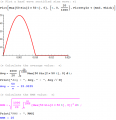Now the calculations for the separate AC and DC parts. The RMS value as calculated in the magenta integral above is 25 volts, the same value we get when we add the AC and DC parts using the correct method; we can't just arithmetically add, we must use the square root of the sum of the squares: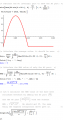#### The Electrician

Joined Oct 9, 2007
2,914
Hi all,

after lots of reading, Googling and ending up getting the same results over and over again, I decided to take a shot and ask the question here.

I'm trying to wrap my head around how a rectified waveform is actually the sum of an AC and DC component. I'm taking an example of a half wave rectifier and I'm talking about the unsmoothed ripple here, so no filter capacitor included.

Let's say I have a maximum secondary voltage Vp2 of 50V, applied to a load resistance of 800 ohms. The resulting maximum current Im is 50V/800, or 62.5 mA.
Idc then equals 62.5/pi (half-wave rectifier) or 19.89 mA. Irms equals 62.5/2 = 31.25 mA.

Now, a rectified current (or voltage) is actually the sum of an an AC current + DC current. Let's say I visualise the above example (see picture). If I understand it correctly, the peak-peak value of the AC component is equal to the peak-value of the rectified wave (62.5 mA in this example). The minimum value of the AC component however is (in this example) -19.89 mA, so if you add the 19.89 mA DC-component to the AC-component, you get a nice rectified result (blue waveform).

View attachment 94487

The way I understand it and cross-checked with examples and books (Principles Of Electronics among one of them), this drawing makes sense.

However, there's also the maths. As stated in various sources and books:

View attachment 94486

Iac, being the rms value of the ripple current.
Now if I make that calculation in my example (sqrt of 31.25² - 19.89²), I get an Iac(rms) of 24.10 mA (as extra proof I calculate the ripple factor Iac(rms)/Idc = 24.10/19.89 = 1.21, which is ripple factor for a half wave rectifier, so this adds up).

However, when I try to calculate the Iac(peak) value out of the rms, I get 24.10 mA x 2 (half wave rectifier), which give me 48.20 mA.
Which is different from the peak value I got when I made the drawing (should be the same

Now I know I'm doing something wrong and since the math works out, it probably is the drawing, but if that is not correct, can someone explain me how exactly the rectified output is a combination of AC and DC? Because when I draw it this way (which somehow seems to make sense, the math doesn't work out?

Thanks!
Here it is worked out for your currents: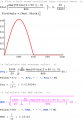And the second part: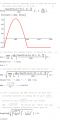As wayneh pointed out, for this waveform it's no longer true that the peak current is equal to 2 times the RMS value. That's your problem.

Last edited:

#### CurlsOnKeys

Joined Jan 3, 2015
47
Here it is worked out for your currents:

Here it is worked out for your currents:

View attachment 94548
Brilliant! Thanks a lot for all your time and efforts!
I'd figured out something similar yesterday night thanks to all the different contributions and new insights to this topic and was planning to post the solutions, but you beat me to itAnyway, here I go, just to add my final thoughts:

Problem:
Suppose we have a rectified waveform (full or half wave), Vout, then this waveform contains an AC component and a DC component. As Peter pointed out, an FFT can split up this AC component in its various frequencies. Add those Fourier-terms back together and you get one AC-waveform. Add the DC component to this one AC-wave and you get your rectified wave. For this to be true, the peak-peak value of the AC-component should be exactly the same as the peak-peak value of your rectified signal (Vout).

Concrete Example:
To be more specific, let's take for example a full rectified wave of 50Vpeak and ignore the diode voltage drops (I know, I said half wave initially, but I eventually figured it out with a full wave)

The average, or DC, of this signal is 2x50V/pi or 31.83 and the RMS is 50V/sqrt(2) or 35.35V.

Now let's suppose, this rectified wave, this Vout is a combination of AC and DC. As Wayneh and MikeML pointed out, to calculate the RMS value of a waveform where the flat part is not at zero, you have to use the integrals. As I found here and as you pointed out aswel, the formula is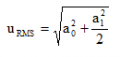. This version of the formula uses the AC-peak value (which, devided by sqrt2 gives the rms value (and becomes the same formula as you used).

Now IF our original assumption was true (rectified = AC + DC), then:

35.35V = sqrt(31.83² + ACpeak²/2), so AC(peak) = sqrt(2x(35.35² - 31.83²) = 21.74V. The ACrms value should then be 21.74/sqrt2 = 15.38V. The ripple factor 15.38V/31.83 = 0.48, so the rms value seems to be correct.

Now here's where I got confused initially: 21.74V AC peak? Why? Shouldn't the peak-value of the AC component be the same as that of the rectified waveform? Where does this 21.74V come from?

So I turned it around: let's assume our AC component HAS the same peak-peak (50V). We've subtracted the DC component from the rectified wave, so the AC component has shifted "downwards" 31.83V.

So now, by using the same formula: ACrms = sqrt(-(31.83)² + 50²/2) = 15.38V = same ACrms as calculated above!

It turns out (of course it does, but I didn't see it), that a single rms value (15.38V in this case) can be the effective value of different waveforms. The initial peak value of 21.74V didn't make any sense (hence my original question), but that's just an AC waveform with the flat part at zero, for which the rms happens to be 15.38V. Those same 15.38 volts however ALSO happen to be the rms for a waveform with a 50V peak and a DC offset of -31.83V, which makes a lot more sense if you draw it, because that's exactly the AC component we've been looking for.

Problem solved, thanks to you all!

@The Electrician : which program did you use to plot the graphs and make the calculations?

•PeterCoxSmith

#### The Electrician

Joined Oct 9, 2007
2,914
•CurlsOnKeys and PeterCoxSmith

#### MikeML

Joined Oct 2, 2009
5,444
I wonder what @The Electrician would have done if @CurlsOnKeys had intended to account for either Ideal or Real rectifier voltage drops. Just because you can do something doesn't mean you should. If you are building something real, using whatever tool gets the job done fastest is what an engineer gets paid for...

#### CurlsOnKeys

Joined Jan 3, 2015
47
I wonder what @The Electrician would have done if @CurlsOnKeys had intended to account for either Ideal or Real rectifier voltage drops. Just because you can do something doesn't mean you should. If you are building something real, using whatever tool gets the job done fastest is what an engineer gets paid for...
I'm not entirely following you here? These are of course theoretical and ideal voltage drops, I'm well aware that voltage drops in reality will be somewhat different. We built a power supply once in the lab and I built several other electronic things: theory is theory, electronic reality is always different. But firmly and thoroughly understanding the (ideal) theory behind the reality helps me when I'm designing and building. That was the intention of this post.

#### PeterCoxSmith

Joined Feb 23, 2015
148
I wonder what @The Electrician would have done if @CurlsOnKeys had intended to account for either Ideal or Real rectifier voltage drops. Just because you can do something doesn't mean you should. If you are building something real, using whatever tool gets the job done fastest is what an engineer gets paid for...
I leaned thing from @The Electrician, Mathematica is a pretty cool mathematics tool, I'm going to buy it!

#### wayneh

Joined Sep 9, 2010
17,189
Another take-home message is to not rely on plug-n-chug formulas. Once I learned calculus way back when, I became leery of using any such formula that I didn't know exactly how it was derived. I always go back to the calculus and derive it myself. That may sound like a pain, and it would be if you haven't made calculus part of your brain, but it's actually quite easy if you keep your calculus skills sharpened. The number of things you need to remember goes WAY down and you see the world differently. A few fundamental rules of physics, when combined with calculus, gives you textbooks full of tools and equations. I just wish I had Mathematica when I was learning this stuff!

•CurlsOnKeys
Totally agree. That was why I kept looking for a proof of this formula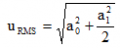. I have to admit calculus is not yet fully "a part of my brain", like multiplying, dividing or other basic maths. Once I see the derivation done, I completely understand it, but doing it myself would take me lots of time (and I'd still doubt if what I'd done was correct). Who knows, one dayThanks all for your help!